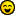# Topic: Calculate Hash Target and Probability in PHP (Read 10556 times)

Activity: 5026
Merit: 11665July 22, 2010, 09:25:42 PM
The difficulty is based on the *minimum* target, and this target is generated rather inexactly as 00000000ffff000000000000000... (eight 0s, four Fs, 0 to taste)

No. That target (which I included in decimal in my second formula) is the maximum allowed target. The target is lowered to increase difficulty. The highest target is the lowest difficulty.

This is because the probability of winning with one hash is target/max. Reducing target reduces the probability of winning.
full memberActivity: 210
Merit: 104July 22, 2010, 08:15:51 PM
"Difficulty" is the number returned by getDifficulty. (The huge number is the maximum target, which getDifficulty is based on.) This calculation is not exact.

I search debug.log to get the target, but the result of the last retarget seems to be less accurate than the calculation above. I'm not sure why this is. Maybe the code changed. (Edit: I now think that this is just an artifact from the "nBits" decompression.)
The difficulty is based on the *minimum* target, and this target is generated rather inexactly as 00000000ffff000000000000000... (eight 0s, four Fs, 0 to taste)
Activity: 5026
Merit: 11665July 17, 2010, 06:41:22 PM
This is how I do probability per hash:
Code:
`\$hash=\$hextarget;\$hash=strtoupper(\$hash);\$probability=`echo "obase=10;ibase=16;scale=37;\$hash/FFFFFFFFFFFFFFFFFFFFFFFFFFFFFFFFFFFFFFFFFFFFFFFFFFFFFFFFFFFFFFFF |bc`;`
(The tickmarks execute the command on the shell.)

Then you do 1/\$probability to get average number of hashes. Divide this by the number of hashes you're getting per second to get the number of seconds.

You can calculate the target like this:
Code:
`26959535291011309493156476344723991336010898738574164086137773096960/difficulty`
"Difficulty" is the number returned by getDifficulty. (The huge number is the maximum target, which getDifficulty is based on.) This calculation is not exact.

I search debug.log to get the target, but the result of the last retarget seems to be less accurate than the calculation above. I'm not sure why this is. Maybe the code changed. (Edit: I now think that this is just an artifact from the "nBits" decompression.)
sr. memberActivity: 294
Merit: 252Firstbits: 1duzyJuly 17, 2010, 03:53:58 AM
The difficulty value is derived from the current target.
You can find the current target by "grep"ing 'After' in the debug.log

Edit: Oh ok, you already know that, nevermind.
hero memberActivity: 574
Merit: 507July 16, 2010, 11:37:09 PM
I am trying to prepare PHP code to calculate exact value for Hash Target similar as to displayed at http://www.alloscomp.com/bitcoin/calculator.php (currently: 1.48501965484E+65).

Can someone help me to refine my code?

`	header("Content-type: text/plain");	require_once 'jsonRPCClient.php';	\$data=new jsonRPCClient('http://127.0.0.1:8332');	\$difficulty = floatval(\$data->getdifficulty());	//     \$a      /         \$b	// (2^256 - 1) / (2^32 * difficulty)	bcscale(256);	\$a = bcsub(bcpow(2,256),1);	//\$a = gmp_strval(gmp_sub(gmp_pow(2,256),1));	\$b = bcmul(bcpow(2,32),\$difficulty);	//\$b = pow(2,32) * \$difficulty;	bcscale(0);	\$target = bcdiv(\$a,\$b);	//\$target = gmp_strval(gmp_div(\$a,\$b));	//\$target = bcdiv(\$a,\$b);	//\$target = "148504231478890412392775945444335243545681910455595839046778120430";	//\$target = "148504231478000000000000000000000000000000000000000000000000000000";	//\$target = "148501965484000000000000000000000000000000000000000000000000000000";	//000000000168fd00000000000000000000000000000000000000000000000000	//         168fcfffffee48119ddbfdc811138960d70605cc300000000000000	\$targethex = gmp_strval(gmp_sub(\$target,0),16);	echo "Current Hash Target: Dec(\$target)    Hex(\$targethex)";?>`
`	bcscale(256);	\$pph = bcdiv(\$target,bcpow(2,256)); // probability per hash	if (isset(\$_GET["r"]) && \$_GET["r"] != "") { // user-defined rate		\$rate = \$_GET["r"];		if (is_numeric(\$rate)) {			function humantime(\$secs) {				if (\$secs<0) return false;				\$m = (int)(\$secs / 60); \$s = \$secs % 60; \$s = (\$s <= 9) ? "0\$s" : \$s;				\$h = (int)(\$m / 60); \$m = \$m % 60; \$m = (\$m <= 9) ? "0\$m" : \$m;				\$d = (int)(\$h / 24); \$h = \$h % 24;				return \$d."d \$h:\$m:\$s";			}			\$pps = bcdiv(bcmul(\$target,1000),bcpow(2,256)); // probability per second			bcscale(16);			\$ppr = bcmul(\$pps,\$rate);			\$etaAvg = humantime(bcdiv(1,\$ppr));			\$eta25 = humantime(bcdiv(-log(.75),\$ppr));			\$eta50 = humantime(bcdiv(-log(.5),\$ppr));			\$eta75 = humantime(bcdiv(-log(.25),\$ppr));			\$eta95 = humantime(bcdiv(-log(.05),\$ppr));			\$eta99 = humantime(bcdiv(-log(.01),\$ppr));			echo "ProbabilityPerSecond(\$ppr) Avg(\$etaAvg) 25%(\$eta25) 50%(\$eta50) 75%(\$eta75) 95%(\$eta95) 99%(\$eta99)";		} else echo "This requires either no argument or a numeric argument representing khash/sec.";	}	else {		bcscale(32);		\$pph = bcmul(\$pph,1);		echo "ProbabilityPerHash(\$pph)";	}?>`